Common Probability Distributions | IFT World
IFT Notes for Level I CFA® Program
IFT Notes for Level I CFA® Program

# Part 1

## 1. Discrete Random Variables

In nearly all investment decisions, we work with random variables. To make probability statements about a random variable, we need to understand its probability distribution. A probability distribution specifies the probabilities of all possible outcomes of a random variable. In this reading, we will look at the following seven probability distributions:

• Uniform
• Binomial
• Normal
• Lognormal
• Student’s t
• Chi-square
• F-distribution

### 1.1 Discrete Random Variables

A random variable is a variable whose outcome cannot be predicted. There are two basic types of random variables:

Solution:

F(1,600) =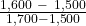= 50% The probability that gold price will be less than $1,600 per ounce three years from now is 50%. ## 3. The Binomial Distribution The building block of the binomial distribution is the Bernoulli random variable. A Bernoulli trial is one where there are only two possible outcomes: success or failure. Flipping a coin is an example of a Bernoulli trial – you either get heads or tails, but nothing else. This can be expressed as: P (Y = 1) = p P (Y = 0) = 1 – p where: p = probability that the trial is a success In a binomial distribution, the random variable, X, is the number of successes in a given number of Bernoulli trials. Continuing with the coin example, say we flip the coin 10 times and we define success as ‘Heads’. Clearly with 10 flips we can get 0 to 10 successes. The probability distribution of a binomial random variable for the probability of “x” success in “n” trials is calculated using the following formula: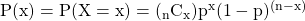where: p = the probability of success on each trial x = number of successes n = number of trials Instructor’s Note: Two important points help illustrate the intuition behind the formula: • The successes can be in any order. That is why we use the combination function and not the permutation function. • The events are independent. That is why we simply multiply the probability of each event. Example If we flip a fair coin (p = 0.5) ten times (n = 10), what is the probability of seven successes? Solution: P(7) = P (X = 7) = 10C7 0.57 0.53 = 0.117 Mean and variance of a binomial variable The mean and variance of a binomial variable can be calculated as:  Random Variable Mean Variance Bernoulli, B (1, p) p p (1 – p) Binomial, B (n, p) np np (1 – p) For our coin-flip example, the mean value of the binomial random variable is np = 10 x 0.5 = 5. The intuition: if we perform the binomial experiment several times, where each experiment refers to 10 coin-flips, on average we will have 5 successes. The actual number of successes will be distributed equally on either side of the mean value. The random value for every trial moves closer to the expected value as the number of trials grows. Example Over the last 10 years, Abro corporation’s EPS increased year over year six times and decreased year over year four times. You decide to model the number of EPS increases for the next decade as a binomial random variable. 1. If success is defined as an increase in the annual EPS, determine the probability of success. 2. What is the probability that EPS will increase in exactly 5 of the next 10 years? 3. Calculate the expected number of yearly EPS increases during the next 10 years. 4. Calculate the variance and standard deviation of the number of yearly EPS increases during the next 10 years. Solution to 1: There are only two possible outcomes: increase in the EPS and no increase in the EPS. Probability of success: p = 6/10 = 0.6 Probability of failure: 1 – p = 1 – 0.6 = 0.4 Solution to 2: Using the binomial model: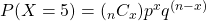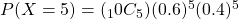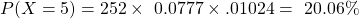Solution to 3: Expected number of yearly EPS increases: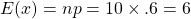Solution to 4: Variance =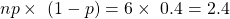Standard Deviation =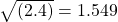The variance of the distribution is calculated as n p (1 – p) = 10 x 0.5 x (1 – 0.5) = 2.5 Binomial tree A binomial tree can be used to model stock price movements. Refer to the tree diagram below. ‘S’ represents the initial stock price. ‘u’ represents an up move and ‘d’ represents a down move. The nodes show each possible value of the stock after 1, 2 and 3 time periods.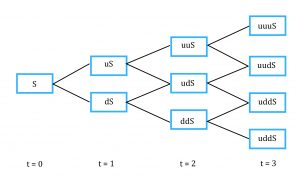The expected stock price after each period is equal to the sum of possible stock prices at the end of the period multiplied by their respective probabilities. Example Consider an initial stock price of$100. In one time period, the stock can either rise by a factor of 1.1 or go down by a factor of 1/1.1. In any given time period, the probability of an up move is 0.6 and the probability of a down move is 0.4. After two periods, what are the possible stock prices and their respective probabilities? What is the expected stock price?

Solution:

uuS = 1.1 x 1.1 x 100 = 121 with probability 0.6 x 0.6 = 0.36

udS = 1.1 x 1/1.1 x 100 = 100 with probability 0.6 x 0.4 = 0.24

duS = 1/1.1 x 1.1 x 100 = 100 with probability 0.4 x 0.6 = 0.24

ddS = 1/1.1 x 1/1.1 x 100 = 82.64 with probability 0.4 x 0.4 = 0.16

Expected stock price = 121 x 0.36 + 100 x 0.24 + 100 x 0.24 + 82.64 x 0.16 = \$104.78